# Translational Motion Projectile Motion Circular Motion Motion Map

• Slides: 22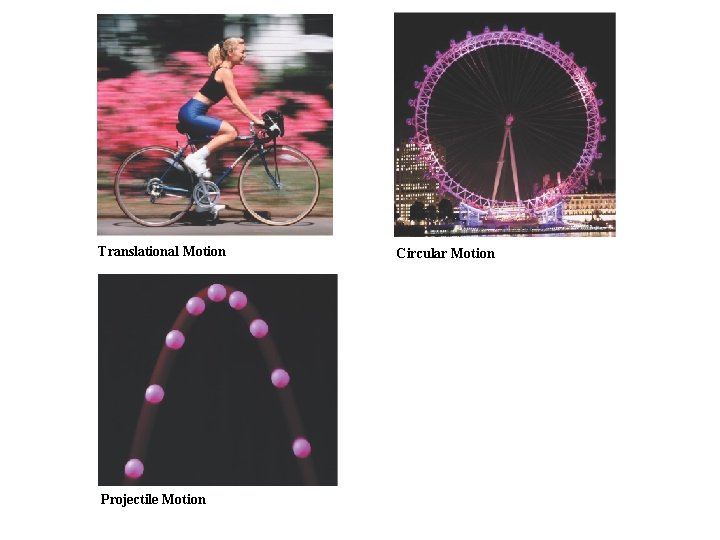Translational Motion Projectile Motion Circular Motion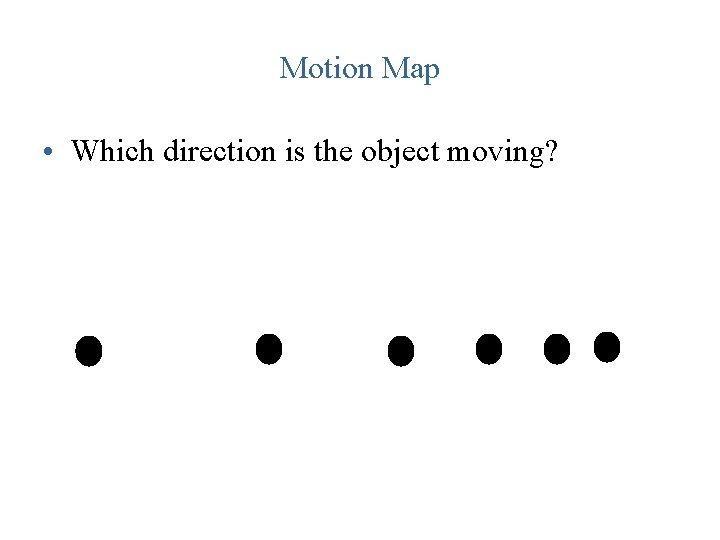Motion Map • Which direction is the object moving?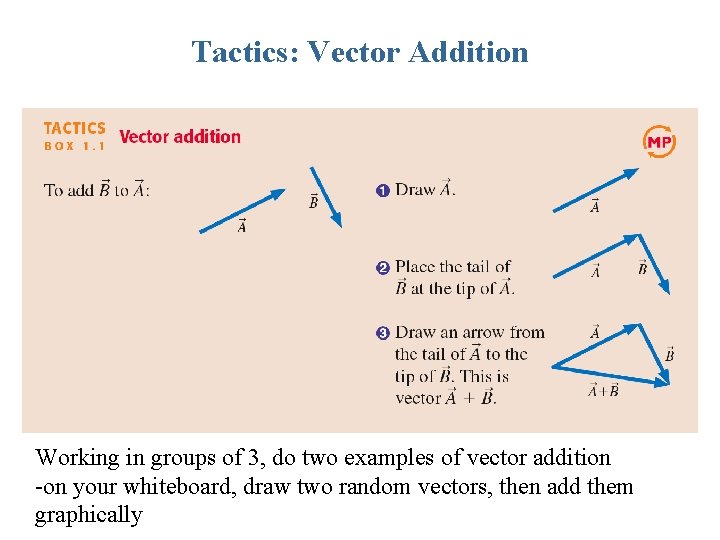Tactics: Vector Addition Working in groups of 3, do two examples of vector addition -on your whiteboard, draw two random vectors, then add them graphically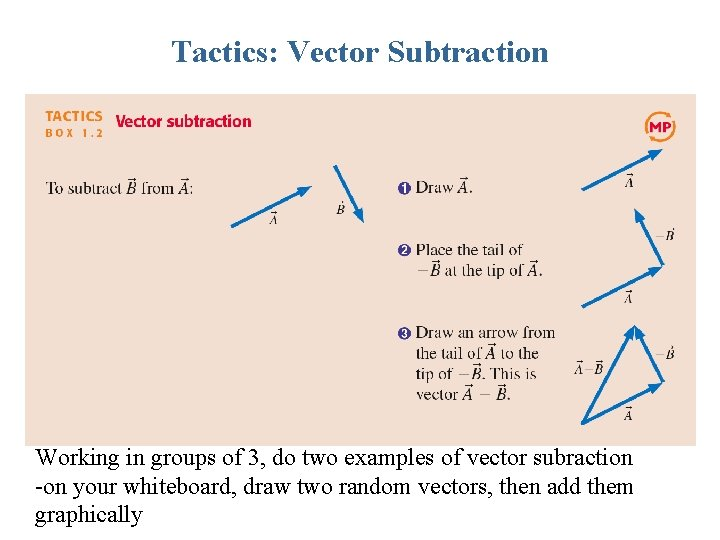Tactics: Vector Subtraction Working in groups of 3, do two examples of vector subraction -on your whiteboard, draw two random vectors, then add them graphically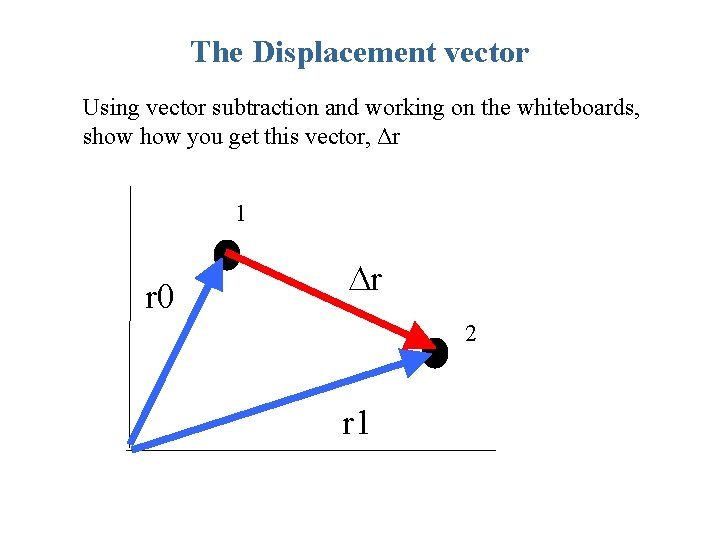The Displacement vector Using vector subtraction and working on the whiteboards, show you get this vector, Δr 1 r 0 Dr 2 r 1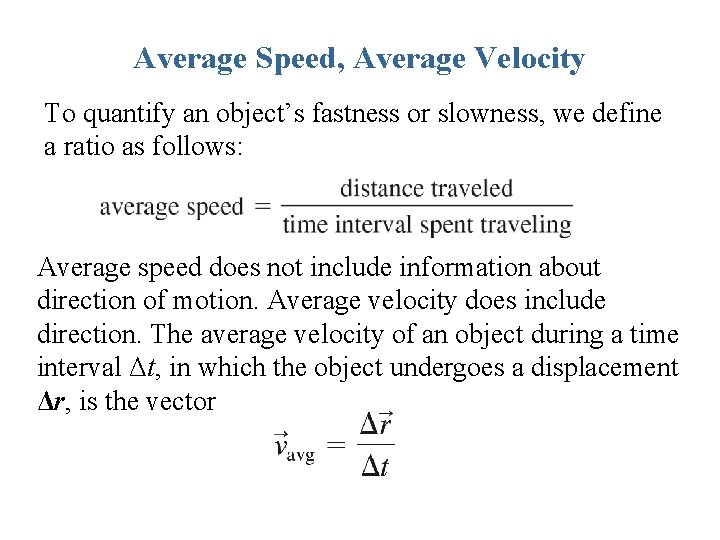Average Speed, Average Velocity To quantify an object’s fastness or slowness, we define a ratio as follows: Average speed does not include information about direction of motion. Average velocity does include direction. The average velocity of an object during a time interval Δt, in which the object undergoes a displacement Δr, is the vector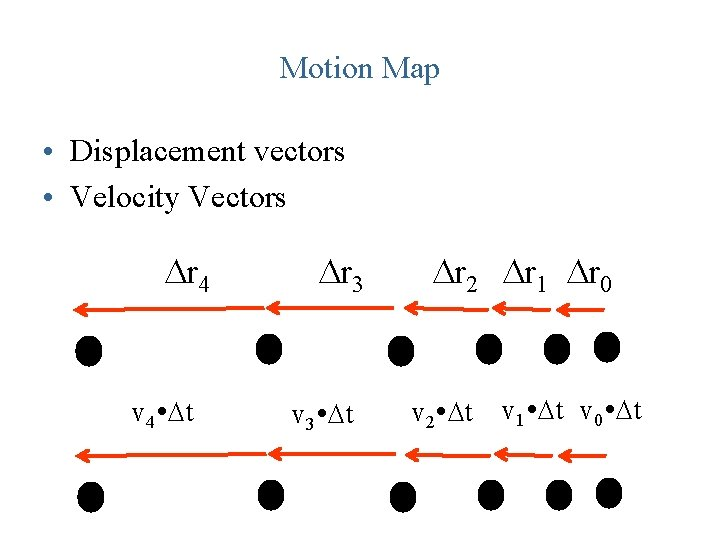Motion Map • Displacement vectors • Velocity Vectors Dr 4 v 4 Dt Dr 3 v 3 Dt Dr 2 Dr 1 Dr 0 v 2 Dt v 1 Dt v 0 DtMotion Map • Δt is the same between each position, so we can drop it • Velocity, v, is proportional to displacement, Δr v 4 v 3 v 2 v 1 v 0Motion Diagrams with Velocity VectorsLinear Acceleration Because velocity is a vector, it can change in two possible ways. 1. The magnitude can change, indicating a change in speed, or 2. The direction can change, indicating that the object has changed direction. We will concentrate for now on the first case, a change in speed.Tactics: Finding the acceleration vector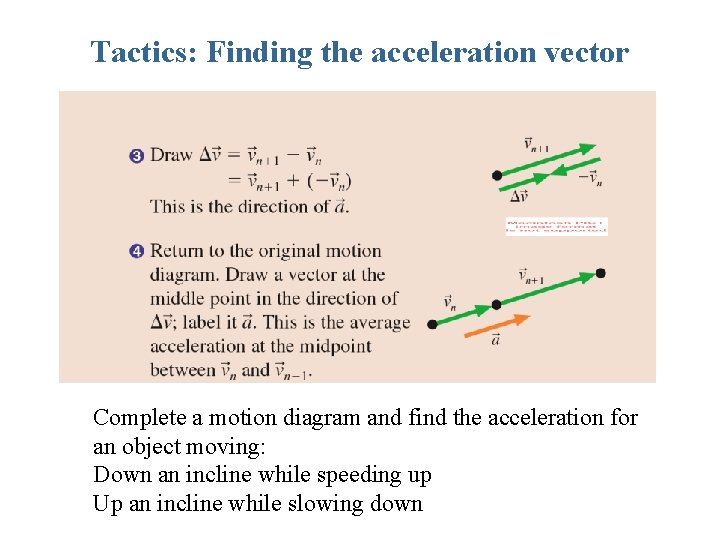Tactics: Finding the acceleration vector Complete a motion diagram and find the acceleration for an object moving: Down an incline while speeding up Up an incline while slowing downA particle undergoes acceleration while moving from point 1 to point 2. Which of the choices shows the velocity vector as the object moves away from point 2?A particle undergoes acceleration while moving from point 1 to point 2. Which of the choices shows the velocity vector as the object moves away from point 2?EXAMPLE 1. 7 Interpreting a position graphEXAMPLE 1. 7 Interpreting a position graph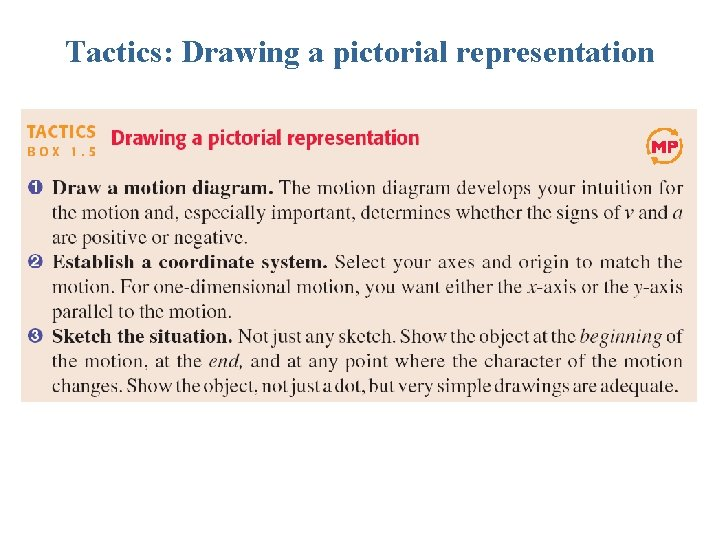Tactics: Drawing a pictorial representation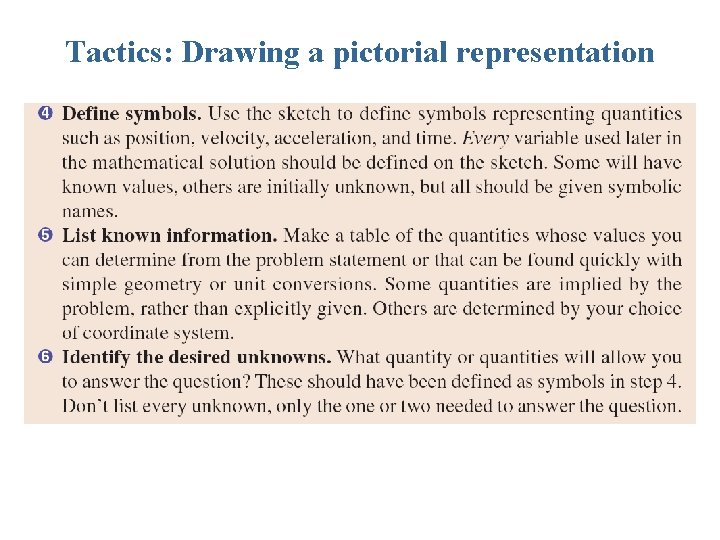Tactics: Drawing a pictorial representation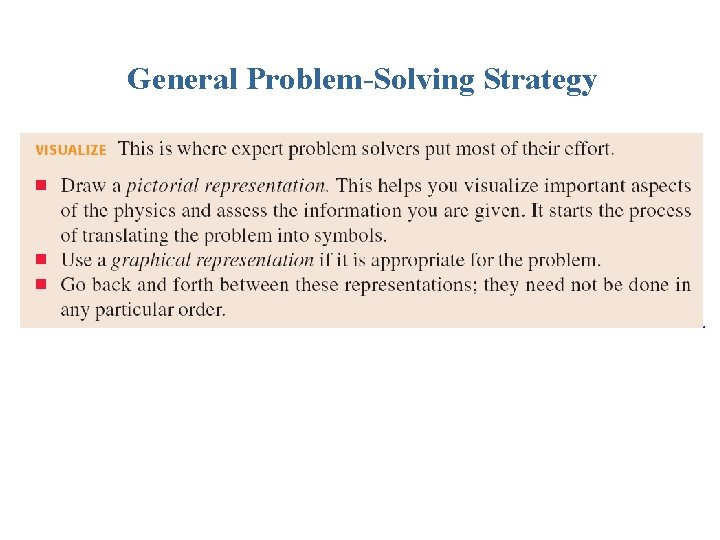General Problem-Solving Strategy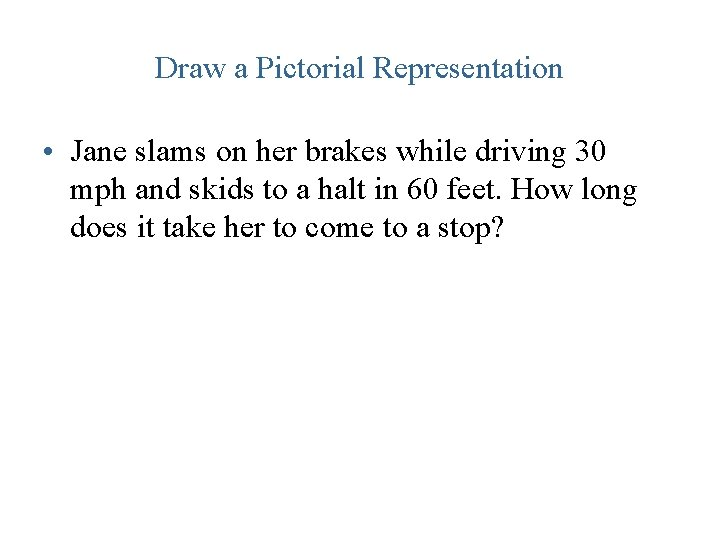Draw a Pictorial Representation • Jane slams on her brakes while driving 30 mph and skids to a halt in 60 feet. How long does it take her to come to a stop?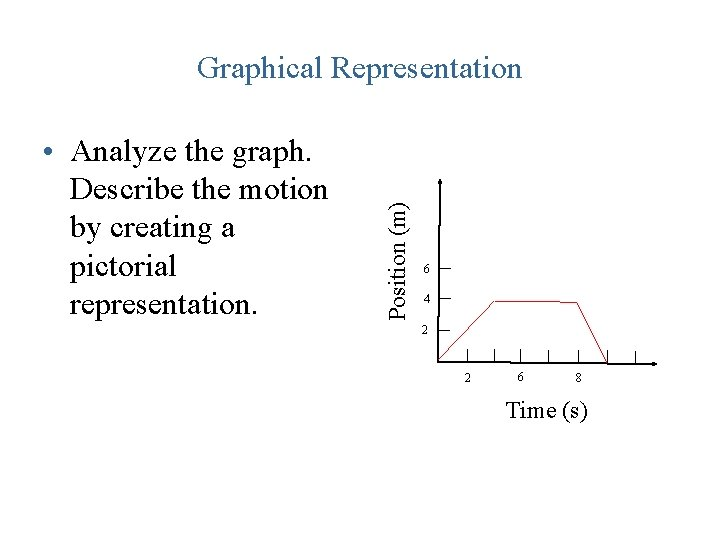• Analyze the graph. Describe the motion by creating a pictorial representation. Position (m) Graphical Representation 6 4 2 2 6 8 Time (s)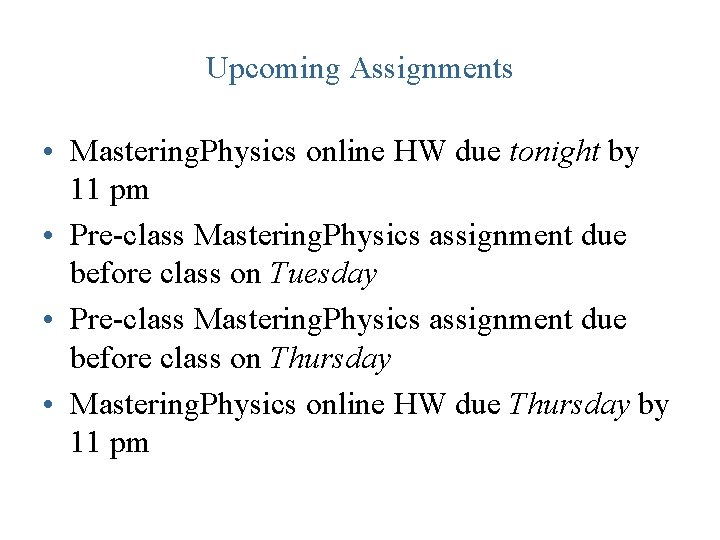Upcoming Assignments • Mastering. Physics online HW due tonight by 11 pm • Pre-class Mastering. Physics assignment due before class on Tuesday • Pre-class Mastering. Physics assignment due before class on Thursday • Mastering. Physics online HW due Thursday by 11 pm